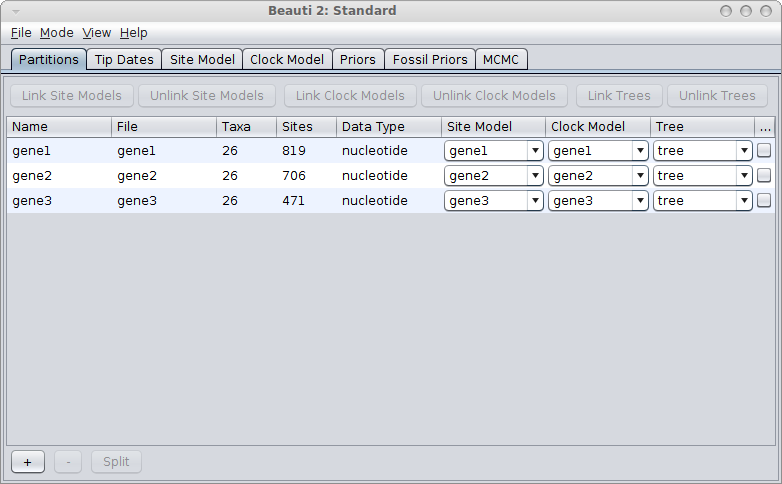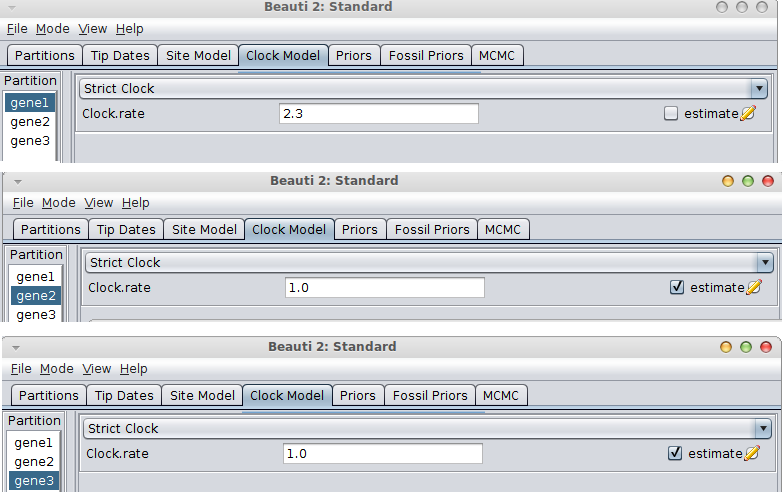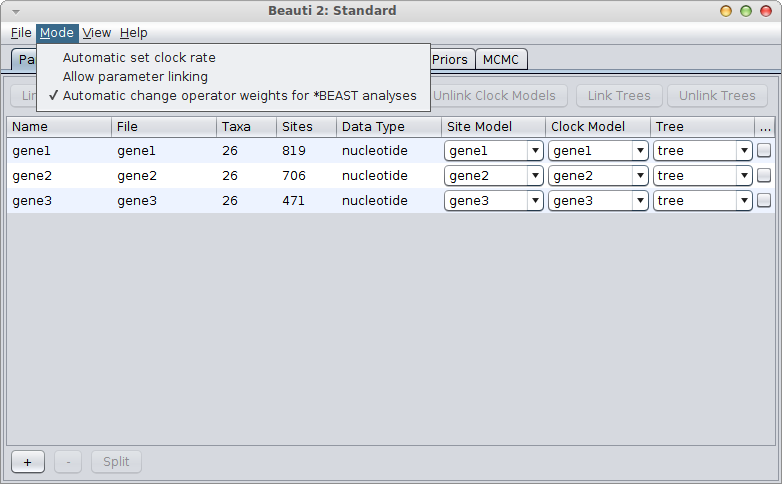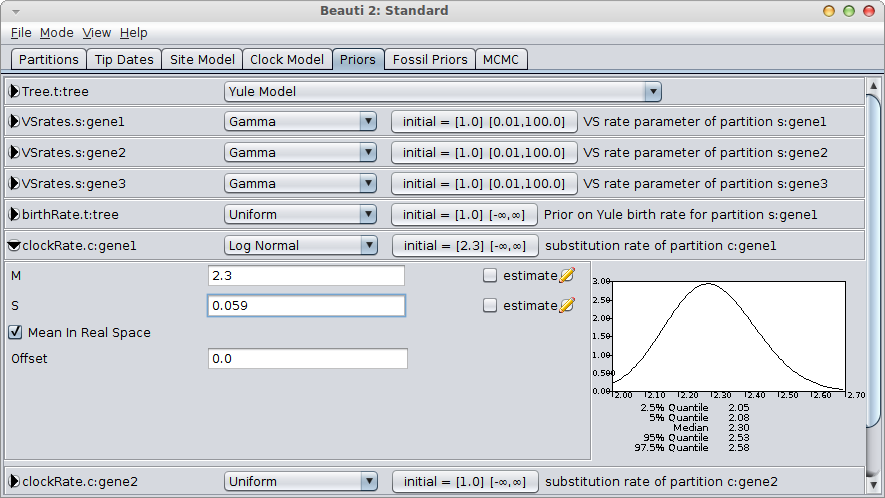# Setting up BEAUti with a known rate

7 July 2014 by Remco Bouckaert

Suppose you have 3 genes and know the rate of one of the genes. How do you set up an analysis in BEAUti?

We call the genes gene1, gene2 and gene3, and the rate is say 2.3 substitutions per site per million year. There are a number of things you can do:

If the genes are linked, for example, because they are all mitochondrial genes, you would use a single tree for all genes. Link trees for all partitions in the partition panel in BEAUti, and it should look something like this:If the rate is known for the first gene, go to the Clock model tab, fill in the clock rate for the gene you have a rate for and BEAUti conveniently fixes the rate for that partition and marks the others as estimated.If the gene with the known rate is not the first partition, you have to uncheck the “Mode/Automatic set clock rates”.Then go to the clock model panel, where you can set the estimate box for each of the genes you want to estimate and uncheck it for the gene with the known rate.

### Independent genes

If all three genes are unlinked, use a *BEAST analysis where you have an independent gene tree for each of your genes. Essentially, the same scenario for clock rates as for the single tree applies.

### Semi-independent genes

You can have a mixture of the above scenarios when two genes are linked and one is independent of the two, say, when two genes are mitochondrial and one nuclear. Then, a *BEAST analysis is more appropriate, with two gene trees — one for the linked genes and one for the other.

## Uncertainty: rate = 2.3 ± 0.25

Often, there is some measure of uncertainty for the known rate, so instead of 2.3, we find 2.3 ± 0.25 in the literature. Then, it would be more appropriate to estimate the known clock rate as well, and put a strong prior on that clock rate. In this case, you want to uncheck the “Mode/Automatic set clock rates” menu and make sure all clock rates have their “estimate” box ticked in the clock model panel. You set the clock-rate prior in the priors panel.

It is tempting to interpret a rate estimate of 2.3 ± 0.25 as a normal distribution with mean of 2.3 and a 95% confidence interval of [2.3-0.25, 2.3+0.25] = [2.05, 2.55]. However, the problem with a normal distribution is that its range includes negative values. In general, if the mean is more than 4 standard deviations away from zero, this can be reasonable, since less than 0.006% of the probability mass is below zero.

### What is wrong with the normal prior?

Even then it is still possible that during the MCMC, the clock rate may become zero (or even negative) and there is no indication from the prior that this is not allowed. This can happen because other priors interact with the rate prior. In particular, the tree priors (e.g. a coalescent when there are enough taxa) can strongly guide the height of the tree, and thus interact with the rate prior. The tree prior should be conditioned on the rate calibration (prior = P(tree|calibration) * P(calibration)), but in BEAST we generally assume (incorrectly) priors are independent (prior = P(tree) * P(calibration)), because we do not know how to do the conditioning. This means, unfortunately, that this interaction is not obvious when sampling from the prior, and when inspecting the trace it seems we are sampling from the normal rate prior that we specified. Only when sampling from the posterior, both priors try to influence the height of the tree, and start interacting with each other.

So, if you insist on using a normal distribution, you must verify that the rate is distributed according to your prior (by inspecting the trace file in Tracer) and the rate does not contain zero or negative values. (You could ensure that the rate remains positive by setting a lower bound on the clock rate).

### Use a log-normal prior!

A much better alternative is to use a log-normal prior, which has a range over the positive numbers only. For small values of the standard deviation, it is shaped like a bell-curve. Just like the normal distribution, it only requires two parameters to specify, but requires no lower bound to be set on the rate, since negative values are automatically out of range.

It is easy to configure the log-normal prior in BEAUti, by setting the mean “M” to 2.3, check the “Mean in real space” box (as opposed to in log space) and set the standard deviation “S” by matching the 95% interval to [2.05, 2.55]. This means matching the 2.5% quantile to 2.05 and the 97.5% quantile to approximately 2.55. A bit of fiddling with the numbers shows that S=0.059 is a reasonable match.You still want to verify that the rate is distributed according to your rate prior (by inspecting the trace file in Tracer) and is not influenced by the tree prior too much.